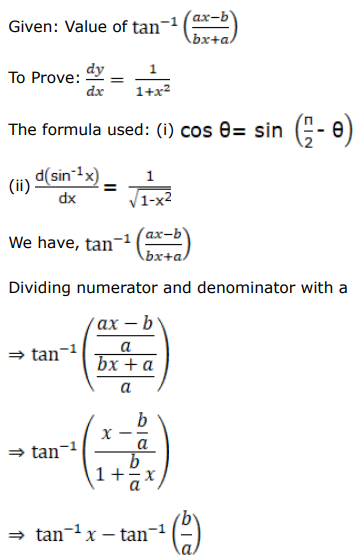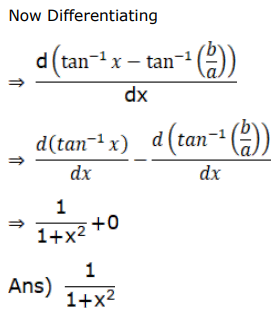# Differentiate each of the following w.r.tQuestion:

Differentiate each of the following w.r.t $x$ :

If $t=\tan ^{-1}\left(\frac{a x-b}{b x+a}\right)$, prove that $\frac{d y}{d x}=\frac{1}{\left(1+x^{2}\right)}$

Solution: